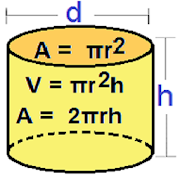## Cylinder Calculator helps you practice Grade HS Math - Lumos Educational App StorePrice -fREE

#### DESCRIPTION:

Cylinder Calculator Use the Cylinder Calculator to calculate height, radius, volume, curved surface area, areas of the top and bottom circles, or total surface area of a cylinder. Length :millimeter (mm); centimeter (cm); meter (m); foot (ft); inch (in) Area : square millimeter (mm^2); square centimeter (cm^2); square kilometer (km^2); square meter (m^2); square foot (ft^2); square inch (in^2) Volume :milliliter (mL); centiliter (cL); liter (L); kiloliter (kL); cubic meter (m^3); cubic centimeter (cm^3); cubic millimeter (mm^3); barrel (US); barrel (UK); gallon (US); gallon (UK); c

#### OVERVIEW:

Cylinder Calculator is a free educational mobile app By .It helps students in grades HS practice the following standards HSG.GMD.A.1.

This page not only allows students and teachers download Cylinder Calculator but also find engaging Sample Questions, Videos, Pins, Worksheets, Books related to the following topics.

1. HSG.GMD.A.1 : Give an informal argument for the formulas for the circumference of a circle area of a circle volume of a cylinder pyramid and cone. Use dissection arguments, Cavalieris principle, and informal limit arguments..

HS

#### STANDARDS:

HSG.GMD.A.1

Software Version: 1

Category:

### RELATED APPS

Rate this App?
0

0 Ratings & 0 Reviews

5
0
0
4
0
0
3
0
0
2
0
0
1
0
0EdSearch WebSearch